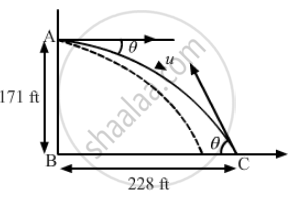Advertisement Remove all ads

# A person standing on the top of a cliff 171 ft high has to throw a packet to his friend standing on the ground 228 ft horizontally away. - Physics

Short Note

A person standing on the top of a cliff 171 ft high has to throw a packet to his friend standing on the ground 228 ft horizontally away. If he throws the packet directly aiming at the friend with a speed of 15.0 ft/s, how short will the packet fall?

Advertisement Remove all ads

#### Solution

Given:
Height (h) of the cliff = 171 ft
Horizontal distance from the bottom of the cliff = 228 ft
As per the question, the person throws the packet directly aiming to his friend at the initial speed (u) of 15.0 ft/s.From the diagram, we can write:

$\tan \theta = \frac{P}{B} = \frac{171}{228}$

$\Rightarrow \theta = \tan^{- 1} \left( \frac{171}{228} \right)$
∴ θ = 37°
When the person throws the packet from the top of the cliff, it moves in projectile motion.
Let us take the reference axis at point A.
u is below the x-axis.
a = g = 32.2 ft/s2 (Acceleration due to gravity)
Using the second equation of motion, we get:

$y = u\sin\left( \theta \right)T + \frac{1}{2}g T^2$

$y = 171 ft$

$\theta = 37^\circ$

$g = 32 ft/ s^2$

$T = \text{ Time of flight }$

$171 = 15\sin\left( 37 \right)T + \frac{1}{2} \times 32 \times T^2$

$\text{ On solving this quadratic equation in T, we get } :$

$T = 2 . 99 s$

$\text{ Range } = 15\cos\left( 37 \right) \times 2 . 99 = 35 . 81 ft$

$\text{ Distance by which the packet will fall short } = 228 - 35 . 81 = 192 . 19 ft$

Is there an error in this question or solution?
Advertisement Remove all ads

#### APPEARS IN

HC Verma Class 11, 12 Concepts of Physics 1
Chapter 3 Rest and Motion: Kinematics
Q 37 | Page 52
Advertisement Remove all ads
Advertisement Remove all ads
Share
Notifications

View all notifications

Forgot password?